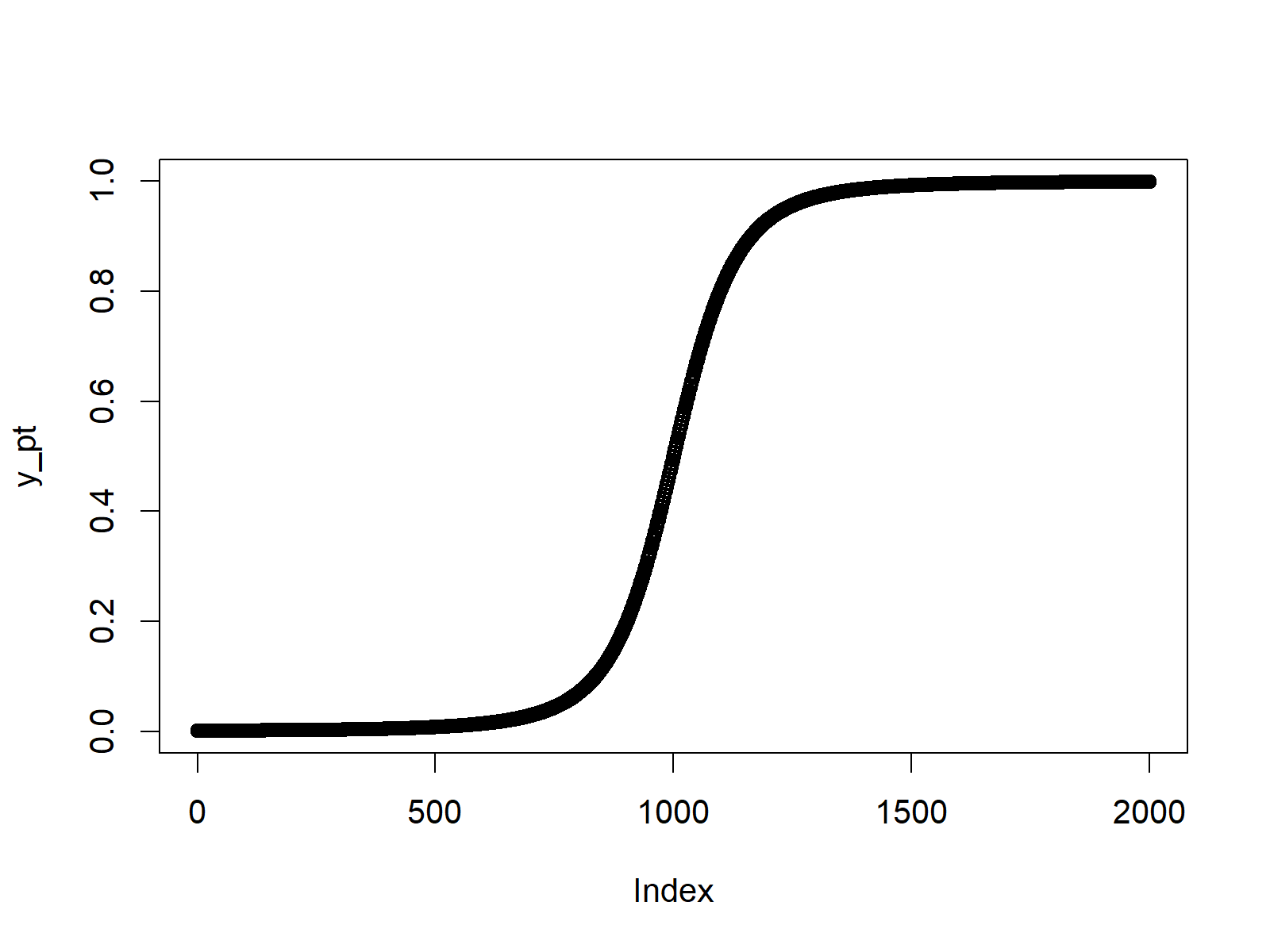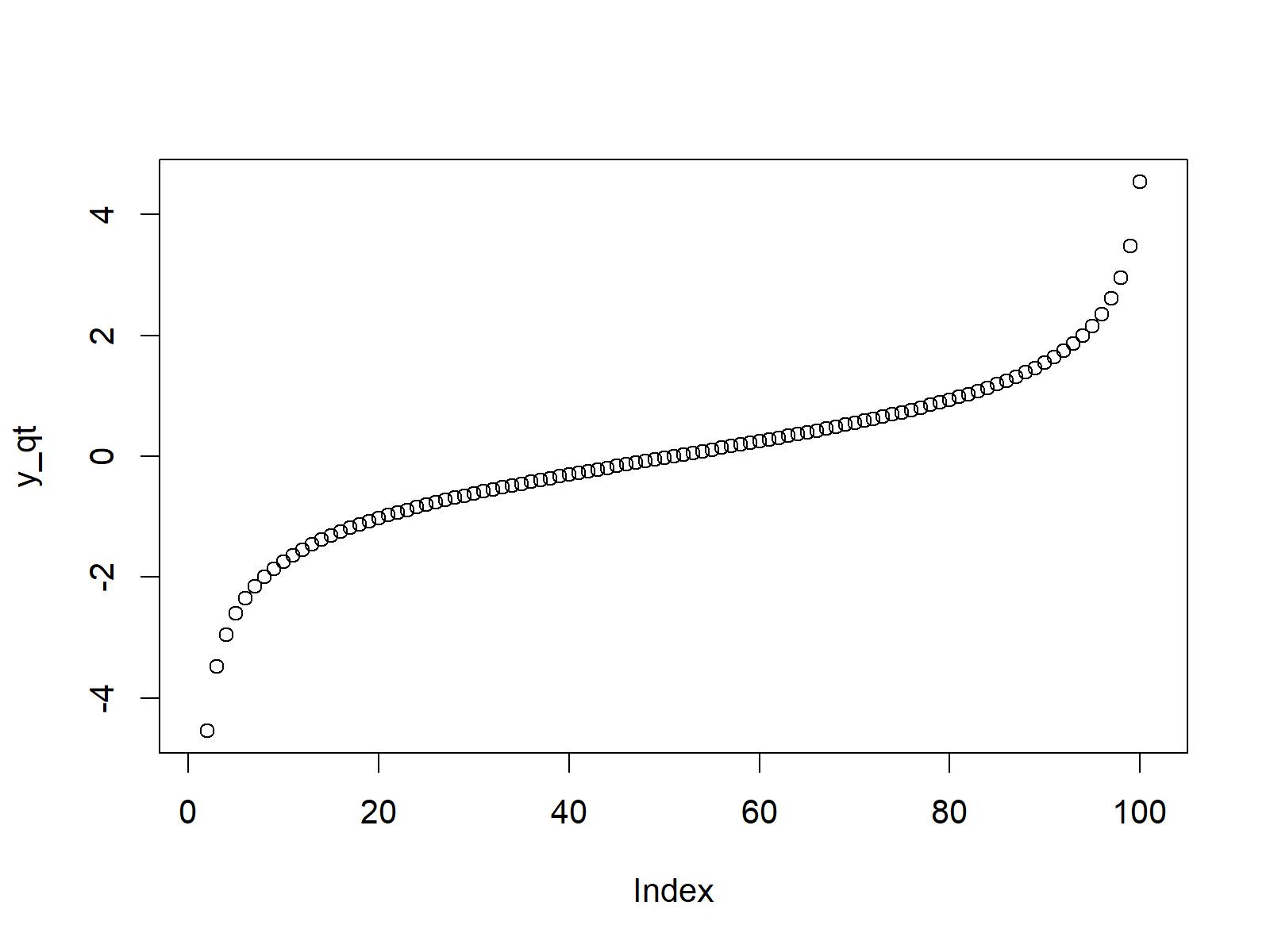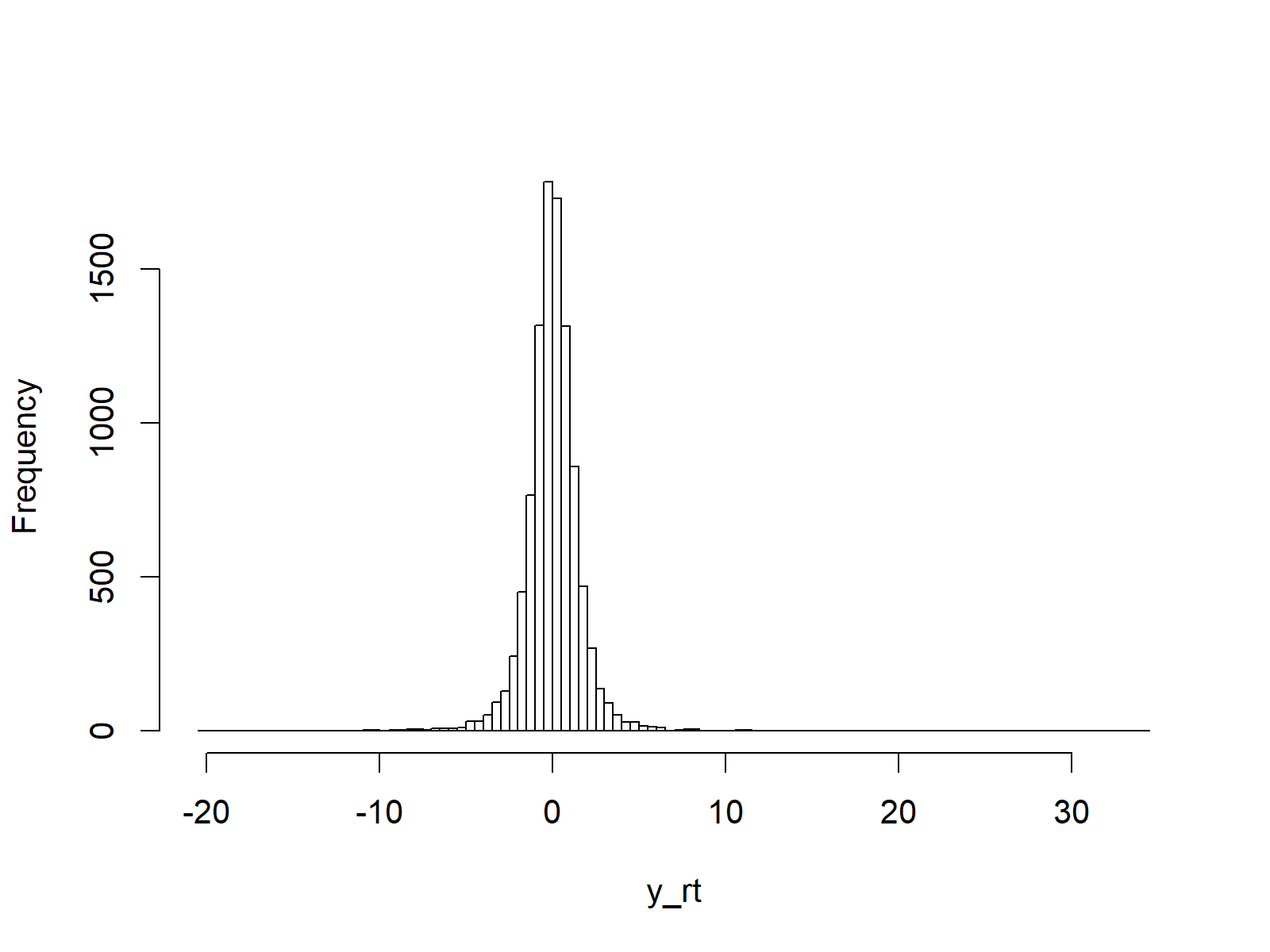# Student t distribution in R (4 Examples) | dt, pt, qt & rt Functions

This article shows how to apply the Student t functions in R.

The tutorial is structured as follows:

Let’s dive right into the examples.

## Example 1: Student t Probability Density Function (dt Function)

In the first example, we’ll create a graphic showing the density of the Student t distribution.

First, we need to create a vector of quantiles in R:

`x_dt <- seq(- 10, 10, by = 0.01)                    # Specify x-values for dt function`

After running the previous R code, we can apply the dt command in R as follows. In the example, we use 3 degrees of freedom (as specified by the argument df = 3):

`y_dt <- dt(x_dt, df = 3)                            # Apply dt function`

The Student t density values are now stored in the data object y_dt. We can draw a graph representing these values with the plot R function:

`plot(y_dt)                                          # Plot dt values`Figure 1: Density of Student t Distribution in R.

## Example 2: Student t Cumulative Distribution Function (pt Function)

This example shows how to draw the cumulative distribution function (CDF) of a Student t distribution.

As in the previous example, we first need to create an input vector:

`x_pt <- seq(- 10, 10, by = 0.01)                    # Specify x-values for pt function`

Then, we can apply the pt function to this input vector in order to create the corresponding CDF values:

`y_pt <- pt(x_pt, df = 3)                            # Apply pt function`

Finally, we can apply the plot function to draw a graphic representing the CDF of the Student t distribution in R:

`plot(y_pt)                                          # Plot pt values`Figure 2: Cumulative Distribution Function of Student t Distribution in R.

## Example 3: Student t Quantile Function (qt Function)

If we want to draw a plot of the quantile function of the Student t distribution, we need to create a sequence of probabilities as input:

`x_qt <- seq(0, 1, by = 0.01)                        # Specify x-values for qt function`

We then can apply the qt R command to these probabilities:

`y_qt <- qt(x_qt, df = 3)                            # Apply qt function`

The corresponding plot can be created with the plot function as follows:

`plot(y_qt)                                          # Plot qt values`Figure 3: Quantile Function of Student t Distribution in R.

## Example 4: Generating Random Numbers (rt Function)

We can also apply the Student t functions in order to generate random numbers. First, we have to set a seed for reproducibility and we also need to specify a sample size N that we want to simulate:

```set.seed(91929)                                     # Set seed for reproducibility
N <- 10000                                          # Specify sample size```

We can now use the rt function to generate our set of random numbers:

```y_rt <- rt(N, df = 3)                               # Draw N log normally distributed values
y_rt                                                # Print values to RStudio console```

The following histogram illustrates the distribution of our random numbers (i.e. a Student t distribution):

```hist(y_rt,                                          # Plot of randomly drawn student t density
breaks = 100,
main = "")```Figure 4: Random Numbers According to Student t Distribution in R.

## Video, Further Resources & Summary

Have a look at the following video of my YouTube channel. In the video tutorial, I’m explaining the contents of this tutorial.

You may also have a look at the other articles on distributions and the simulation of random numbers in R:

Furthermore, I can recommend to read the related tutorials on this website. Please find a selection of interesting articles here.

In this article you learned how to draw and simulate a Student t distribution in the R programming language. Please let me know in the comments below, in case you have any further questions.

Subscribe to the Statistics Globe Newsletter